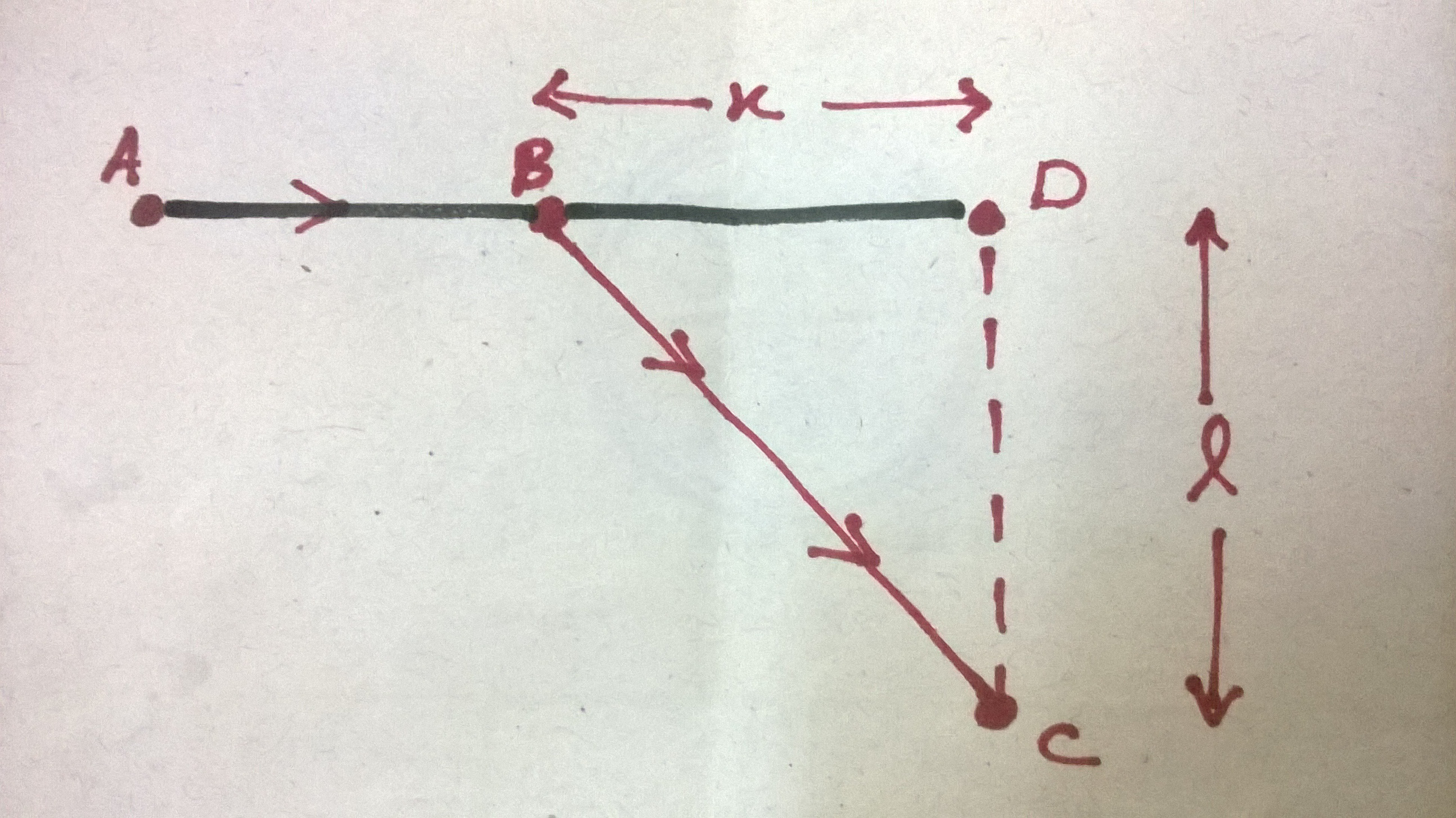# Solve it without calculus or quadraticA person starts walking from point $A$ with a certain velocity on the highway. Point $C$ is in the grassland at a distance $l$ from the highway and he wants to get there as soon as possible. However, his speed is $Q$ times slower walking on grass than on highway. At what distance $x$ $(AD>x)$ from point $D$ should he change his path so that he reaches point $C$ in minimum time.

Assume $l=9\text{ m}$, $Q= 10^{\frac{1}{2}}$.

×